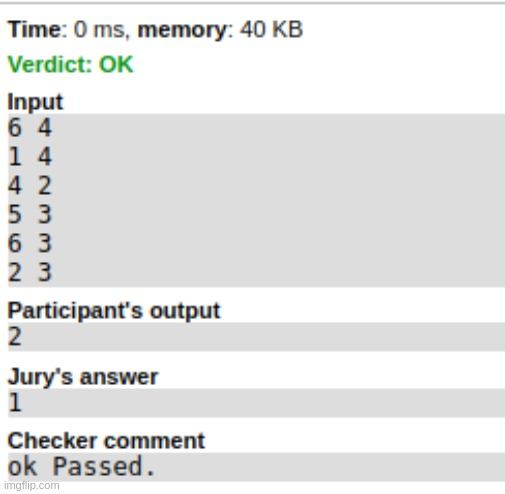### fugazi's blog

By fugazi, history, 13 months ago,I was solving this Problem.

The root (supposed to be unique) is the second number in the first line.

But the checker shows different answer, and eventhough my answer is different than checker it still passes.

Or is it that checker is outputting something else ? like number of queries used or something?

My codeRead more »

By fugazi, history, 21 month(s) ago,My friend gave me a problem, which can be reduced to the following.

Let $S(k)$ be the set of all arrays of size $n$ that contains $k$ ones and $n - k$ zeroes. Let for some array $s \in S(k)$ , $pos_s[1..k]$ denotes the position of those ones (say in increasing order, it doesn't matter actually). We have to calculate.

$\sum_{s \in S(k)} {\sum_{i = 1}^{k} pos_s[i] }$

i.e, for all arrays $s \in S(k)$ we have to sum the positions of all $k$ ones.

My thoughts : Modification to binomial expansion? Noo. hmm, what about counting how many times each position occurs, but then there will be intersections, like I might put two ones at the same index, so ? what about inclusion-exclusion? No it's getting a lot messy.

Linearity of Expectation : Let's find the expected value of $\sum_{i = 1}^{k} pos_s[i]$

$E[\sum_{i = 1}^{k} pos_s[i]]$

Let's look at it it some other way. We choose random variables $X_{1, 2, .. k}$ lying in $[1, n]$ with same probability (Note that I'm not saying they're independent. ). Thus $E[X_i] = \frac{1 + 2 + .. n}{n} = \frac{n + 1}{2}$ Now by linearity of expectation,

$E[\sum_{i = 1}^{k} X_i] = \sum_{i = 1}^{k} E[X_i] = \sum_{i = 1}^{k} \frac{n + 1}{2} = \frac{k(n + 1)}{2}$

.

Now, it's left to you to realise that

$E[\sum_{i = 1}^{k} pos_s[i] = E[\sum_{i = 1}^{k} X_i]$

.

And since we've $n \choose k$ arrays possible, we deduce,

$\sum_{s \in S(k)} {\sum_{i = 1}^{k} pos_s[i] }= \frac{{n \choose k} k (n + 1)}{2}$

.

I went numb for a few seconds after completing the solution, I mean wtf, what about intersection, how can this be even possible, you can't just choose it randomly (I know the formal proof of LOE, but still :\ )

There are few things, that I know are true, but I can never digest them, "My favourite song has just 7 million views on youtube" is on second", "Linearity of expectation actually works" remains at first.

Maybe I overkilled it. Other easier solutions are welcome :)

Read more »By fugazi, history, 23 months ago,I'm getting WA on codeforces, while its working correctly for that case on my system and even on codeforces custom test. Also the checkers comment is too weird.

Can't find file K:\invoker-prod\work\codeforces6\25bd73a20640963248bd7f3788f1424e\check-1b3eb285945d2168cb2ee5ad536c2f21\run\output.txt [invokerId=7b1ec022e70f30d35dabadbce49e1a1e, location=203279216] (1).

Anybody knows what this might mean? code

Read more »

By fugazi, history, 23 months ago,I am getting a very strange bug in my code for today's round problems B1, 2.

At a moment, I'm having a set of pairs.

[s]: {(1, 1), (1, 4), (1, 5), (1, 9), (1, 10), (2, 2), (2, 7)}

Then I'm accessing the last and second last element. (line 51-54 in mycode)

it = s.rbegin();  // prints *it = (2, 7)
it--;
// prints *it = (1, 10)


But for some weird reason it jumps off (2, 2) and I'm unable to figure out why.

Any help will be appreciated.

Read more »#c++,
By fugazi, history, 3 years ago,I was setting up topcoder applet, but for that I needed to have java environment installed and enabled in browser. But chromium and firefox doesn't support java plugin :( . Which browser you people use then. Or am I missing something?Read more »